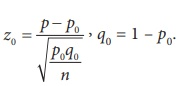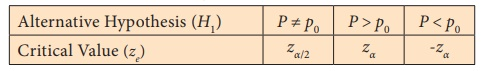Home | | Statistics 12th Std | Test of Hypotheses for Population Proportion

# Test of Hypotheses for Population Proportion

General Procedure for Test of Hypotheses for Population Proportion: Procedure Steps, Example Solved Problems

TEST OF HYPOTHESES FOR POPULATION PROPORTION

## Procedure:

Step 1 : Let P denote the proportion of the population possessing the qualitative characteristic (attribute) under study. If p0 is an admissible value of P, then frame the null hypothesis as H0:P = p0 and choose the suitable alternative hypothesis from

(i) H1: P ≠ p0 (ii) H1: P > p0 (iii) H1: P < p0

Step 2 : Let p be proportion of the sample observations possessing the attribute, where n is large, np > 5 and n(1 – p) > 5.

Step 3 : Specify the level of significance, α.

Step 4 : Consider the test statistic Zunder H0. Here, Q = 1 – P.

The approximate sampling distribution of the test statistic under H0 is the N(0,1) distribution.

Step 5 : Calculate the value of Z under H0 for the given data asStep 6 : Choose the critical value, ze, corresponding to α and H1 from the following tableStep 7 : Make decision on H0 choosing the suitable rejection rule from the following table corresponding to H1.### Example 1.12

A survey was conducted among the citizens of a city to study their preference towards consumption of tea and coffee. Among 1000 randomly selected persons, it is found that 560 are tea-drinkers and the remaining are coffee-drinkers. Can we conclude at 1% level of significance from this information that both tea and coffee are equally preferred among the citizens in the city?

### Solution:

Step 1 : Let P denote the proportion of people in the city who preferred to consume tea.

Then, the null and the alternative hypotheses are

Null hypothesis: H 0 : P = 0.5

i.e., it is significant that both tea and coffee are preferred equally in the city.

Alternative hypothesis: H 1 : P 0.5

i.e., preference of tea and coffee are not significantly equal. It is a two-sided alternative hypothesis.

Step 2 : Data

The given sample information are

Sample size (n) = 1000. Hence, it is a large sample.

No. of tea-drinkers = 560

Sample proportion (p) = 560/1000 = 0.56

Step 3 : Level of significance

α= 1%

Step 4 : Test statistic

Since n is large, np = 560 > 5 and n(1 – p) = 440 > 5, the test statistic under the null hypothesis, is Z =.

Its sampling distribution under H0 is the N(0,1) distribution.

Step 5 : Calculation of Test Statistic

The value of Z can be calculated for the sample information fromThus, z0 = 3.79

Step 6 : Critical value

Since H1 is a two-sided alternative hypothesis, the critical value at 1% level of significance is zα/2 = z0.005 = 2.58.

Step 7 : Decision

Since H1 is a two- sided alternative, elements of the critical region are determined by the rejection rule |z0| ≥ z e. Thus it is a two-tailed test. Since |z0| = 3.79 > ze = 2.58, reject H0 at 1% level of significance. Therefore, there is significant evidence to conclude that the preference of tea and coffee are different.

Tags : Procedure Steps, Example Solved Problems | Statistics , 12th Statistics : Chapter 1 : Tests of Significance - Basic Concepts and Large Sample Tests
Study Material, Lecturing Notes, Assignment, Reference, Wiki description explanation, brief detail
12th Statistics : Chapter 1 : Tests of Significance - Basic Concepts and Large Sample Tests : Test of Hypotheses for Population Proportion | Procedure Steps, Example Solved Problems | Statistics